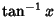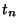## Gregory Number

A numberwhereis an Integer or Rational Number,is the Inverse Tangent, andis the Inverse Cotangent. Gregory numbers arise in the determination of Machin-Like Formulas. Every Gregory numbercan be expressed uniquely as a sum ofs where thes are Størmer Numbers.

References

Conway, J. H. and Guy, R. K. Gregory's Numbers'' In The Book of Numbers. New York: Springer-Verlag, pp. 241-242, 1996.